# LLL basis reduction method

Basis reduction is the process of transforming an arbitrary basis of a lattice into a reduced basis, where a reduced basis consists of short vectors, or, equivalently, a basis that has a small orthogonality defect. Various notions of reduced basis have been proposed by Ch. Hermite [a3], and by A. Korkin, G. Zolotarev and H. Minkowski in the language of quadratic forms (cf. also Geometry of numbers; Quadratic form). A.K. Lenstra, H.W. Lenstra and L. Lovász [a5] introduced a notion of reduced lattice basis together with an efficient algorithm that transforms a given lattice basis into a reduced basis of the same lattice. This algorithm has found numerous applications in number theory and integer optimization, and various cryptographic schemes have been broken using this algorithm.

Letbe the-dimensional real vector space with inner product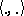and the Euclidean norm. A lattice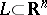is a discrete additive subgroup of(cf. Group). Its rank or dimension is the dimension of the smallest linear subspace that contains. A latticeof rankhas a basis, that is, a set oflinearly independent vectors, so thatconsists of all linear integer combinations of the basis vectors,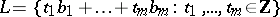.

Let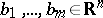be a basis ofwith basis matrix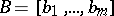consisting of the column vectors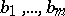. Then all basis matrices ofare of the form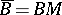, whereis an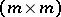integer matrix with determinant.

With an ordered basisone can associate the Gram–Schmidt orthogonalization, which can be computed, together with the Gram–Schmidt coefficients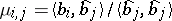, by the recursion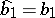,for(cf. also Orthogonalization method). A lattice basisis LLL-reduced if

1)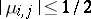for;

2) for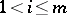,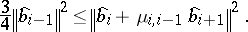For every LLL-reduced basis the length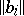approximates theth successive minimumof, where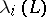is the smallest radius of a ball centred at the origin that containslinearly independent lattice vectors. Always:The novelty of LLL-reduction is a polynomial-time algorithm that transforms an arbitrary integer lattice basis into an LLL-reduced basis [a5]. This algorithm has numerous applications.

For example, polynomials with integer coefficients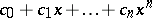can be factored in polynomial time into irreducible factors [a5]. There is a polynomial-time algorithm which, given a rational point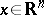and an integer lattice basis, finds a lattice pointthat is nearest toup to a factor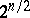[a1]. Linear inequalities with integer coefficients invariablescan be solved over the integers in time, whereis the maximal bit length of the entries in the inequalities [a4], [a6]. In particular,-solutions of a single equation with integer coefficients can be found quite efficiently [a2]. Univariate polynomial equations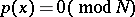with an arbitrary integer moduluscan be solved in polynomial time for small solutions, i.e., solutionswith.

Some applications of LLL-reduction are possible because LLL-reduction can be refined to block reduction so that every reduced basis contains a vector that is not longer than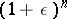times the shortest lattice vector, whereis fixed [a8], [a9]. Block reduction strongly reduces blocks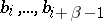of the basis with fixed size. LLL-reduction is the case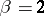. The running time of block-reduction increases withasapproaches. Shortest lattice vectors of lattices of dimension up to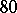can be found within a few hours running time. LLL-reduction can be extended to reduce a set of linearly dependent real vectors. One can derive from LLL-reduction a continued fraction algorithm for arbitrary dimension [a4].